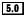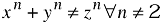# Equation

## Name

Equation -- A displayed mathematical equation

## Synopsis

### Content Model

```Equation ::=
((Title,TitleAbbrev?)?,
(InformalEquation|
(Alt?,
(Graphic+|MediaObject+))))```

### Attributes

Common attributes

 Name Type Default Label CDATA None

### Tag Minimization

Both the start- and end-tags are required for this element.

### Parameter Entities

 %admon.mix; %bookcomponent.content; %component.mix; %divcomponent.mix; %footnote.exclusion; %formal.class; %formal.exclusion; %glossdef.mix; %highlights.exclusion; %para.mix; %qandaset.mix; %refcomponent.mix; %sidebar.mix; %tbl.table.excep;

## Description

An Equation is a formal mathematical equation (with an optional rather than required title).

### Processing expectations

Formatted as a displayed block. For an inline equation, use InlineEquation.

Processing systems that number equations or build a table of equations at the beginning of a document may have difficulty correctly formatting documents that contain both Equations with Titles and Equations without Titles. You are advised to use InformalEquation for equations without titles.

###Future Changes

In some future version of DocBook, probably V5.0, even though the change has not yet been announced, the Title on Equation will be required. For equations without titles, use InformalEquation.

### Children

The following elements occur in Equation: Alt, Graphic, InformalEquation, MediaObject, Title, TitleAbbrev.

In some contexts, the following elements are allowed anywhere: BeginPage, IndexTerm.

## Attributes

Label

Label specifies an identifying string for presentation purposes.

Generally, an explicit Label attribute is used only if the processing system is incapable of generating the label automatically. If present, the Label is normative; it will used even if the processing system is capable of automatic labelling.

## Examples

```<!DOCTYPE equation PUBLIC "-//OASIS//DTD DocBook V3.1//EN">
<equation><title>Fermat's Last Theorum</title>
<alt>x^n + y^n &ne; z^n &forall; n &ne; 2</alt>
<graphic fileref="figures/fermat"></graphic>
</equation>```

Equation 1. Fermat's Last Theorum